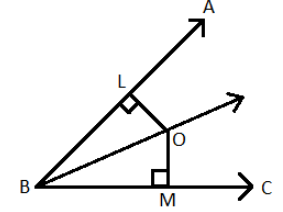QUESTION

# In the figure given below, OL is perpendicular to AB and OM is perpendicular to BC such that OL = OM. Is ∆OLB ≌ ∆OMB?Hint: Let us firstly learn about Congruent Triangles.
CONGRUENT TRIANGLES: Congruent Triangles are triangles that have the same size and shape. This means that the corresponding sides are equal as well as the corresponding angles are also equal.
We can prove that two triangles are congruent by 4 ways: SSS, SAS, ASA and RHS.

GIVEN: OL = OM
OL is perpendicular to AB
OM is perpendicular to BC
TO PROVE: ∆OLB ≌ ∆OMB
PROOF: In ∆OLB and ∆OMB,
OL = OM (Given in the question)
∆OLB and ∆OMB are right angled triangles. (Given in the question)
OB = OB (Common)
Therefore, ∆OLB ≌ ∆OMB (By RHS Congruence Rule)
NOTE:- Let us now learn about the different rules by which we can prove that any two triangles are congruent.
SIDE – SIDE – SIDE (SSS): If three sides of one triangle are equal to three sides of another triangle, then the two triangles are congruent by SSS Congruence Rule.
SIDE – ANGLE – SIDE (SAS): If two sides and the angle between the two sides of one triangle are equal to two sides and the angle between the two sides of the other triangle, then the two triangles are congruent by SAS Congruence Rule.
ANGLE – SIDE – ANGLE (ASA): If two angles and the side between the angles of one triangle are equal to two angles and the side between the angles of another triangle, then the two triangles are congruent by ASA Congruence Rule.
RIGHT ANGLE – HYPOTENUSE – SIDE (RHS): In two ‘right – angled’ triangles, if the length of the hypotenuse and one side of one triangle is equal to the length of the hypotenuse and one side of the other triangle, then the two triangles are congruent by RHS Congruence Rule.